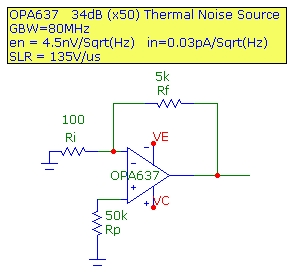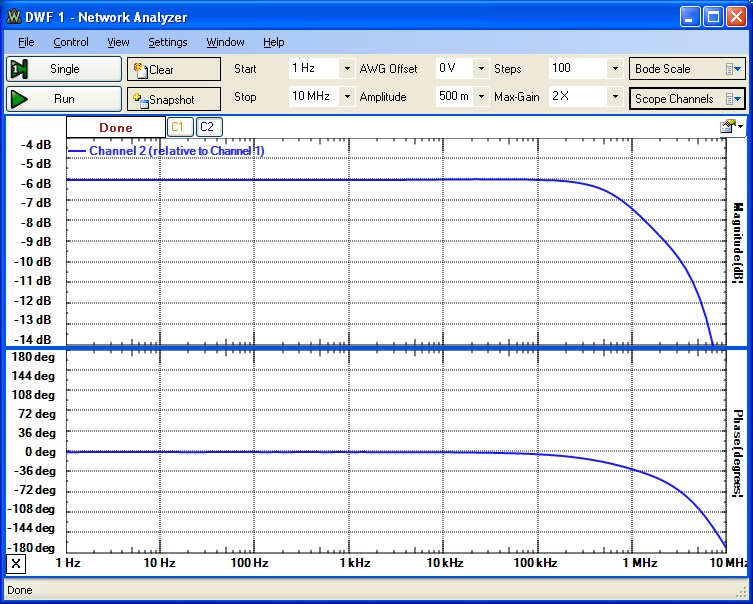# Op-Amp Thermal Noise Source

July 14, 2013

The circuit below demonstrates a simple approach to generating a flat thermal-noise spectrum up to ~ 100 kHz for audio testing purposes. The op-amp and circuit components are chosen so that the output noise will be dominated by the thermal noise for high values of Rp. For this purpose, a FET input op-amp is used which has very low current and voltage noise spectral densities. A simple non-inverting amplifier configuration is used with a closed-loop gain of 50 (34 dB). The bandwidth for this circuit with this gain and the OPA637BP op-amp is measured to be ~ 2 MHz as shown below. Since the thermal noise of a resistor in this frequency range will be quite flat and characterized by the Johnson spectral noise density, this provides a useful noise source with a reasonably well characterized noise value with a total noise value in the 100 uVrms range. (The total input capacitance for this op-amp including some parasitic capacitance will be ~ 15 pF at the noninverting input in parallel with Rp so this will in fact cause the thermal noise due to Rp to be filtered at the input above ~ 200 kHz for Rp=50kohm.). Since the nominal offset voltage at room temperature of this op-amp is ~ 100uV, the output offset voltage for this circuit will be < 5mV:Total output rms voltage noise measurements were obtained for a measured noise bandwidth of 46kHz using a very low-noise audio sound card sampling at 96kHz. The measured total rms output noise values are shown compared with the calculated output rms noise (assuming constant spectral voltage and current noise densities of the op-amp). The result for Rp=50kohm is dominated by the thermal noise of Rp. For Rp=100 ohm (or shorted) the output noise is dominated by the op-amp voltage noise (4.5nV/Sqrt(Hz)). In both cases, the measured results are in very good agreement with the calculated results:
```
Rp(ohm)              Measured               Calculated            Calculated Input-Referred
---------           -------------           -------------         ---------------------------
50 kohm              320 uV                    310 uV                        6.2 uV

100 ohm               51 uV                     52 uV                        1.0 uV

```

A simple protoboard circuit is shown below which will give stable and low-noise performance over the frequency range of interest if placed in a shielded box as shown:## Frequency Response

The Bode plot below shows the frequency response of the circuit at an input signal level of 5mVp (250mVp output) demonstrating a -3db BW of ~ 2 MHz. (The -6dB level shown results from the measurement configuration which included an attenuator between the scanned reference signal source and the noise-circuit input):